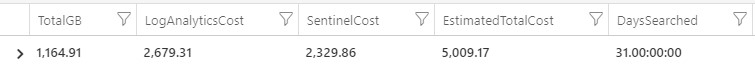# Azure Sentinel – Costing Estimate (PAYG)

In this example, now that Azure Sentinel is Generally Available (GA) we can look at the Azure Monitor Logs (Log Analytics) and Azure Sentinel charges. I have used Pay as You Go (PAYG) for both, using USD \$ and EASTUS as the region, but please feel free to adapt to you local region or currency. You need data in your Azure Monitor Logs workspace for this to work.

Also see
https://azure.microsoft.com/en-gb/pricing/calculator/

Example Query

``` // Disclaimer: All prices shown are in USD (\$). This is a summary estimate, not a quote. // source: https://azure.microsoft.com/en-us/pricing/ // Clive Watson Microsoft // let daystoSearch = 31d; // Please enter how many days worth of data to look at? let ala_payg = 2.30; // Azure Log Analytics Pricing (\$ USD EASTUS PAYG) let sen_payg = 2.00; // Azure Sentinel Pricing (\$ USD EASTUS PAYG) union withsource = tt * | where TimeGenerated > startofday(ago(daystoSearch)) and TimeGenerated < startofday(now()) // Only look at chargable Tables | where _IsBillable == True | summarize TotalGBytes =round(sum(_BilledSize/(1024*1024*1024)),2), EstimatedALA_USD=round(ala_payg * sum(_BilledSize/(1024*1024*1024)),2), EstimatedSEN_USD=round(sen_payg * sum(_BilledSize/(1024*1024*1024)),2), OldestRecord =min(TimeGenerated), NewestRecord=max(TimeGenerated) by Solution=tt | sort by TotalGBytes desc // stop query here if you want a row by row breakdown (per Table) | summarize TotalGB =sum(TotalGBytes), LogAnalyticsCost =sum(EstimatedALA_USD), SentinelCost =sum(EstimatedSEN_USD), EstimatedTotalCost=sum(EstimatedALA_USD + EstimatedSEN_USD), DaysSearched =daystoSearch ```

——————————————————————————————————————————-

You can comment out or just run the lines above the final “summarize”, if you’d like to see a row by row breakdown of the data.

Run the above on our demo data here

Example outputI’m looking to add the retention and Capacity Reservation – would that be of interest?

Thanks Clive
Find me on https://techcommunity.microsoft.com/t5/Azure-Log-Analytics/bd-p/AzureLogAnalytics